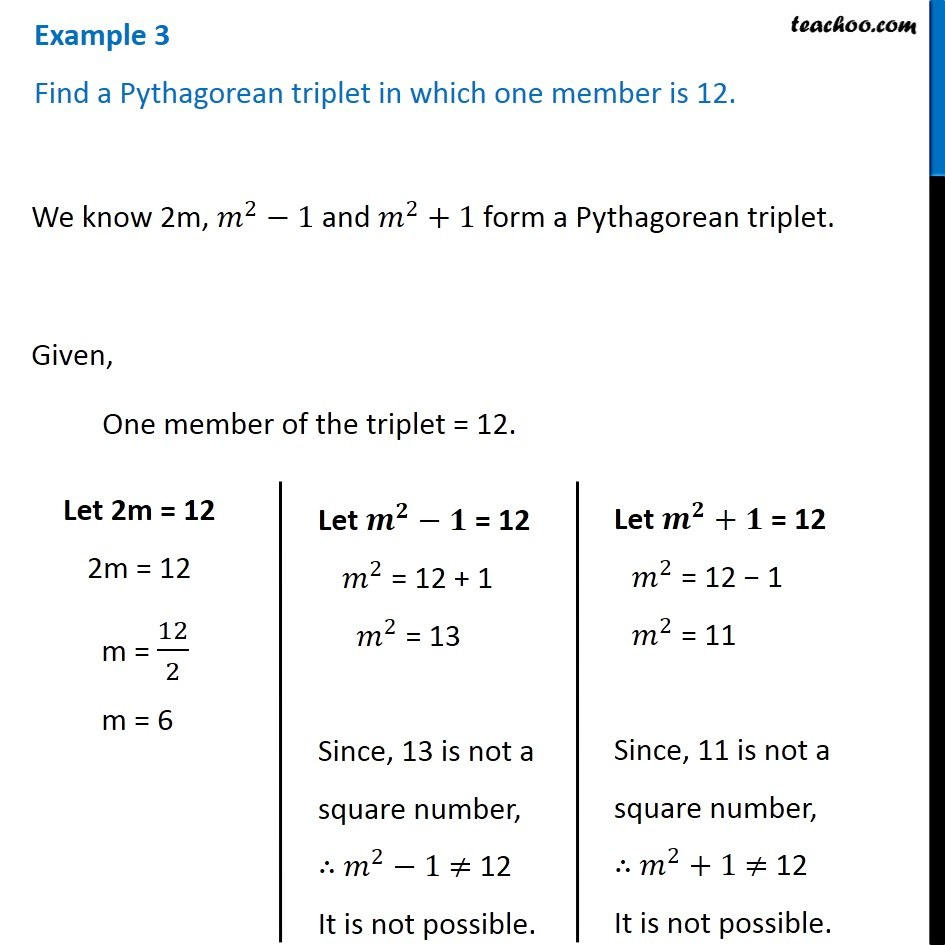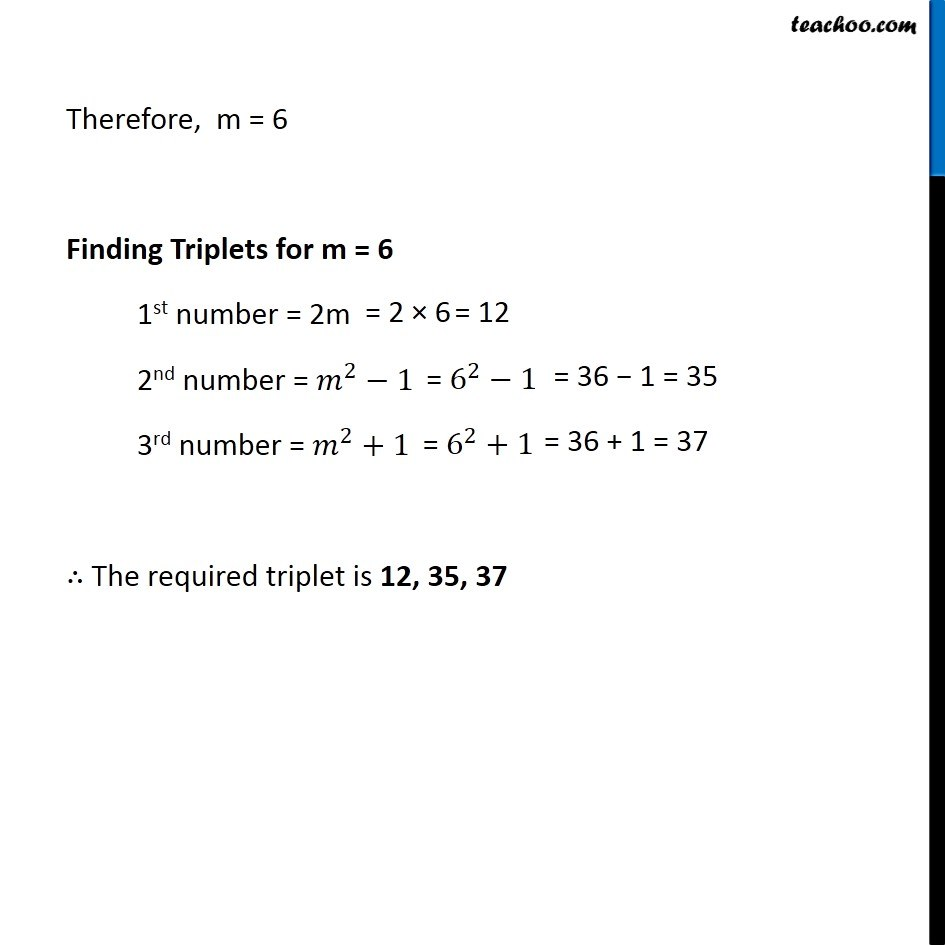Subscribe to our Youtube Channel - https://you.tube/teachoo

1. Chapter 6 Class 8 Squares and Square Roots
2. Concept wise
3. Pythagorean triplets

Transcript

Example 3 Find a Pythagorean triplet in which one member is 12. We know 2m, 𝑚^2−1 and 𝑚^2+1 form a Pythagorean triplet. Given, One member of the triplet = 12. Let 2m = 12 2m = 12 m = 12/2 m = 6 Let 𝒎^𝟐−𝟏" = 12" 𝑚^2 = 12 + 1 𝑚^2 = 13 Since, 13 is not a square number, ∴ 𝑚^2−1 ≠ 12 It is not possible. Let 𝒎^𝟐+𝟏 = 12 𝑚^2 = 12 − 1 𝑚^2 = 11 Since, 11 is not a square number, ∴ 𝑚^2+1 ≠ 12 It is not possible. Therefore, m = 6 Finding Triplets for m = 6 1st number = 2m 2nd number = 𝑚^2−1 3rd number = 𝑚^2+1 ∴ The required triplet is 12, 35, 37

Pythagorean triplets

About the AuthorDavneet Singh
Davneet Singh is a graduate from Indian Institute of Technology, Kanpur. He has been teaching from the past 9 years. He provides courses for Maths and Science at Teachoo.